Custom SearchCHAPTER 8 COMBINATIONS AND PERMUTATIONS LEARNING OBJECTIVES Upon completion of this chapter, you should be able to do the following: 1. Define combinations and permutations. 2. Apply the concept of combinations to problem solving. 3. Apply the concept of principle of choice to problem solving. 4. Apply the concept of permutations to problem solving. INTRODUCTION This chapter deals with concepts required for the study of probability and statistics. Statistics is a branch of science that is an outgrowth of the theory of probability. Combinations and per� mutations are used in both statistics and probability; and they, in turn, involve operations with factorial notation. Therefore, combinations, permutations, and factorial notation are dis�cussed in this chapter. DEFINITIONS A combination is defined as a possible selection of a certain number of objects taken from a group without regard to order. For instance, suppose we were to choose two letters from a group of three letters. If the group of three letters were A, B, and C, we could choose the letters in combinations of two as follows: AB, AC, BC The order in which we wrote the letters is of no concern; that is, AB could be written BA, but we would still have only one com�bination of the letters A and B. A permutation is defined as a possible selection of a certain number of objects taken from a group with regard to order. The permutations of two letters from the group of three letters would be as follows: AB, AC, BC, BA, CA, CB The symbol used to indicate the foregoing combination will be ,C, meaning a group of three objects taken two at a time. For the previous permutation we will use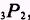, meaning a group of three objects taken two at a time and ordered. You will need an understanding of factorial notation before we begin a detailed discussion of combinations and permutations. We define the product of the integers n through 1 as n factorial and use the symbol n! to denote this; that is,EXAMPLE: Find the value of 5! SOLUTION:EXAMPLE: Find the value of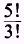SOLUTION: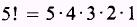and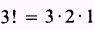Then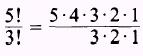and by simplification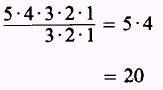The previous example could have been solved by writing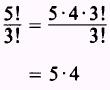Notice that we wrote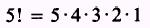and combine the factorsas 3! so thatEXAMPLE: Find the value ofSOLUTION: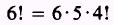and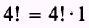Then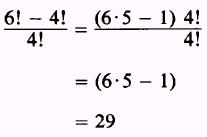Notice that 4! was factored from the expression 6! - 4! Theorem. If n and r are positive integers, with n greater than r2 then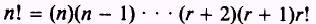This theorem allows us to simplify an expression as follows:Another example is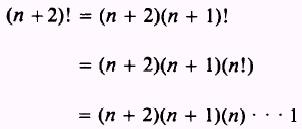EXAMPLE: Simplify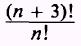SOLUTION:thenPRACTICE PROBLEMS: Find the value of problems 1 through 4 and simplify problems 5 and 6.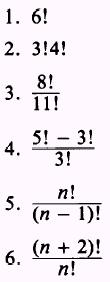ANSWERS: